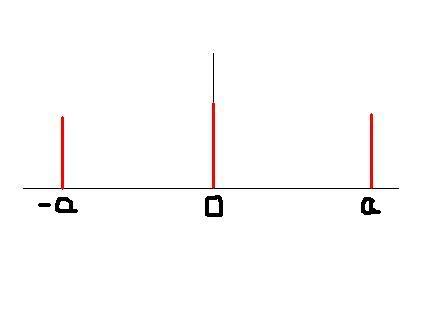# Is this a graph of a delta function?

## Homework Statement

My question asks me to sketch the following:
$$g(x) = \delta (y+a) + \delta (y) + \delta (y-a)$$

## The Attempt at a SolutionI think this is it, but am I correct? I don't recall actually seeing a delta function other than a Kronicker(sp?) delta function, and I'm pretty sure that this isn't one of that type. Research on the web therefore leads me to this (because after trawling my notes I can't actually find anything about it in the entire course!).

Well, except that those aren't infinitely tall! Actually, I don't think that is a very good question because the "delta function" isn't a true "function" and doesn't have a graph. A rough "physicist's" idea of the graph of a delta function $\delta(x)$ would be an infinitely high vertical line at x= 0. Since this is in "precalculus", your three (infinitely high) vertical lines at -a, 0, and a are what I would guess are intended here.